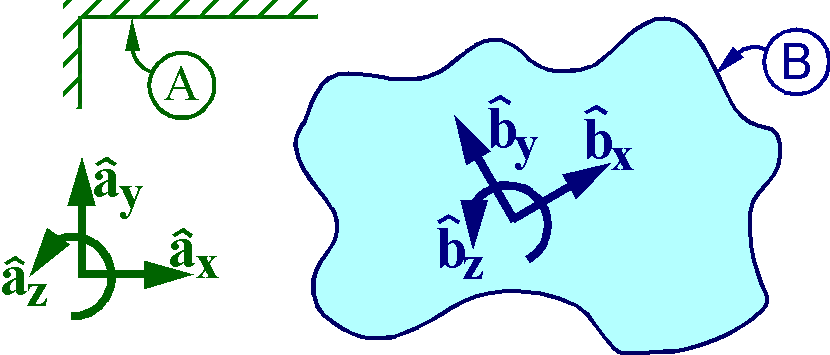Vectors and vector commands

The greater-than symbol   >   denotes vectors.
For example, to multiply 2 by the   zero vector   and assign the result, type
`twoTimesZeroVector> = 2 * 0>`
To create sets of right-handed orthogonal unit vectors:
Ax>, Ay>, Az>   fixed in a reference frame A, and
Bx>, By>, Bz>   fixed in a rigid body B, type
``` RigidFrame A
RigidBody  B ```
Subsequently, other vectors may be defined in terms of these unit vectors, e.g.,
```v> = 2*Ax> + 3*Ay> + 4*Az>
w> = 6*Ax> + 7*Ay> + 8*Az>   % or  w> = Vector( A, 6, 7, 8] )
F> = 3*Bx> + 5*By> + 7*Bz>   % or  F> = Vector( B, [3, 5, 7] ) ```Input only: VectorSampleCommands.txt Input and output: VectorSampleCommands.html
To multiply the vector   v>   by 5, type
```vFive> = 5 * v>
```

To add vectors   v>   and   w>,   type

```addVW> = v> + w>
```

To dot-multiply   v>   with   w>,   type
Note: Since   dotVW   is a scalar, it does not end with the   >   symbol.

```dotVW = Dot( v>, w> )
```

To cross-multiply   w>   with   v>   and form   w> x (w> x v>)

```crossWV> = Cross( w>, v> )
crossWWV> = Cross( w>,  Cross( w>, v> ) )
```

To determine the   magnitude   and   magnitude-squared   of   v>,   type

```magV = GetMagnitude( v> )
magVSquared = GetMagnitudeSquared( v> )
```

To form the unit vector in the direction of   v>,   type

```unitV> = GetUnitVector( v> )
```

To find the radian-measure of the angle between   v> and   w>,   type

```angleBetweenVW = GetAngleBetweenVectors( v>, w> )
```

To form the ordinary time-derivative of the vector  t*v> + sin(t)*w>,  in reference frame A, type

```vectorDerivative> = Dt( t*v> + sin(t)*w>, A  )
```

To form a rotation matrix relating   Bx>, By>, Bz>   to   Ax>, Ay>, Az>, (in terms of time t)   type

```B.RotateZ( A, t )
```

To express vector v> in terms of   Bx>, By>, Bz>,   (overwrites v>), type

```Express( v>, B )
```

To express vector   v> + F>   in terms of   Ax>, Ay>, Az>,   type

```sumInTermsOfA> = Express( v> + F>,  A )
```

To form the   Bx>, By>, Bz>   measures of w> (without changing w>), type

```wMatrix = Vector( B, w> )
```

 To save input (for subsequent re-use), type: Save VectorSampleCommands.txt To save input and output responses, type: Save VectorSampleCommands.html

Exit the program by typing  Quit.